# Catch up on the latest AI articles# Generating A Variable Simulator For Automated Driving: DriveGAN!

3 main points
✔️ Create a DIFFERENTIAL simulation that can control the agent
✔️ Propose DriveGAN to generate Dynamics Simulator
✔️ Succeeded in generating highly accurate simulators with evaluation metrics such as FVD and Action Consistency

written by Seung Wook KimJonah PhilionAntonio TorralbaSanja Fidler
(Submitted on 30 Apr 2021)
Comments: Published as CVPR 2021 Oral.

Subjects: Computer Vision and Pattern Recognition (cs.CV); Robotics (cs.RO)code：## outline

What does it take for automated driving to be on public roads? In recent years, it has become possible to test self-driving cars on public roads, but there are still some shortcomings, such as the recent Waymo case where the car drove the wrong way because one lane was under construction ( Waymo self-driving taxi confused by traffic cones flees help). cones flees help). Because of the dangers of conducting experiments on public roads and the physical limitations of weather conditions and the number of vehicles, simulators are being used for experiments. In the past, such simulators were created manually, but by using machine learning, it is possible to create a scalable simulator by learning changes in the environment according to behavior directly from data.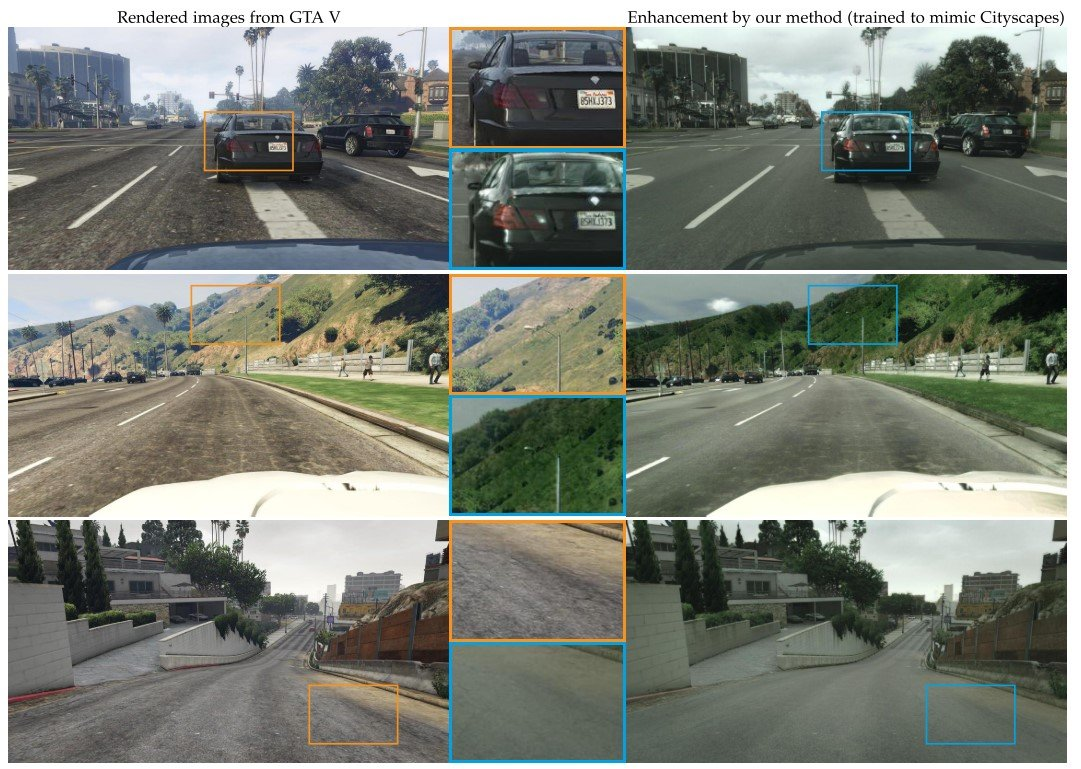Figure 1: Proposed controllable neural simulator, DriveGAN.

Therefore, in this research, we use machine learning techniques to generate a neural simulator that can be used to demonstrate automated driving. We propose DriveGAN, which learns to simulate a dynamic environment directly from pixel space by observing a sequence of unattached frames and their corresponding behaviors. DriveGAN obtains controllability by unsupervised disentangling of different components (i.e., mapping from image space to latent space and then to semantic space, which can be viewed as independent sets). In addition to the control by the handle, the features of a particular scene can be sampled, and it is possible to control the weather and the position of objects.

Since DriveGAN is a fully differentiable simulator, the agent can reproduce the same scene when given a sequence of videos, and can even perform different actions within the same scene (re-simulation). In addition, DriveGAN uses Variational-Auto Encoder and Generative Adversarial Networks to learn the latent space of the image, just as the dynamics engine learns the transitions in the latent space.

### What is Neural Simulator?

The neural simulator learns to simulate the environment according to the agent's behavior directly from pixel space by organizing video data with the behavior. method.

## About DriveGAN

The aim of this study is to learn a high-quality controllable neural simulator by observing a sequence of videos and the corresponding actions. driveGAN acquires controllability in two main aspects.

1. The existence of a self-agent that can be controlled by a given action.
2. Ability to control different possible points such as changing the color of the current scene object or background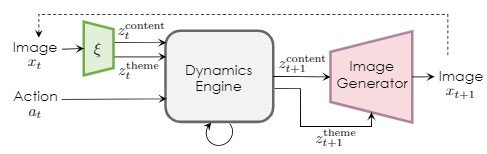Figure 2: Overall view of DriveGAN

We will set up the variables used in the following. First, let $x_t$ be a video frame at time $t$ and $a_t$ be a sequence of actions; the video frame $x_{1:t}$ and actions $a_t$ from the 1st to the tth second are learned to generate the next frame $x_{t+1}$. It generates the disentangled latent variables $Z^{theme}$ and $Z^{content}$ by unsupervised learning. Here, theme refers to information that is independent of background color or weather pixel information, and content refers to elements of space.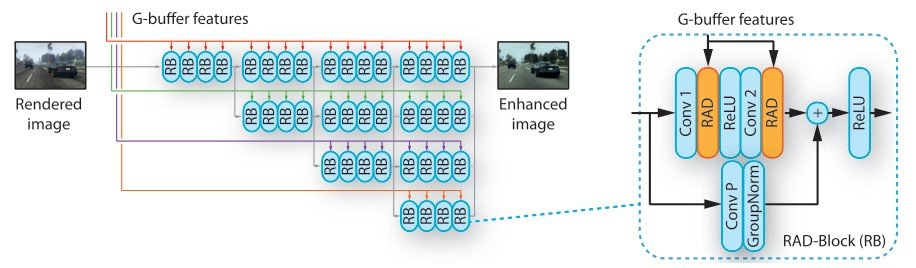Figure 3: Changes in theme information.

The Dynamics Engine consists of a recurrent neural network and generates the latent variables of the next step from the current latent variables and actions. Then, the generated latent variables of the next step are made into the output image using the image decoder.

In this research, we have succeeded in generating more accurate time-series image sequences with reference to the World Model. Next, we describe the structure of the encoder-decoder that is pre-trained to project images into the latent space.

### Pre-trained Latent Space

DriveGAN uses StyleGAN 's image decoder and slightly improves it for theme-content disentanglement. Since it is not trivial to extract the GAN latent variables for the input image, we explicitly project the image $x$ to the latent variable $z$ using encoder $\xi$. Here, we use $\beta$-VAE to control the Kullback Leibler term in the loss function.

We train the generator by adding the following VAE loss functions based on StyleGAN adversarial loss.

\begin{equation} L_{VAE} = E_{z \sim q(z|x)}[\log (p(x|z))] + \beta KL (q(z|x)||p(z)) \end{equation}

Here, $p(z)$ is the standard prior distribution, $q(z|x)$ is the approximate posterior distribution using encoder $\xi$, and KL represents the Kullback-Leibler information content. In the term representing the reconstruction error, we learn to reduce the PERCEPTUAL DISTANCE between the input and output images, not the distance between pixels.

In order to create a controllable simulation, we devised an encoder and decoder.

Disentangle the theme and content of the input image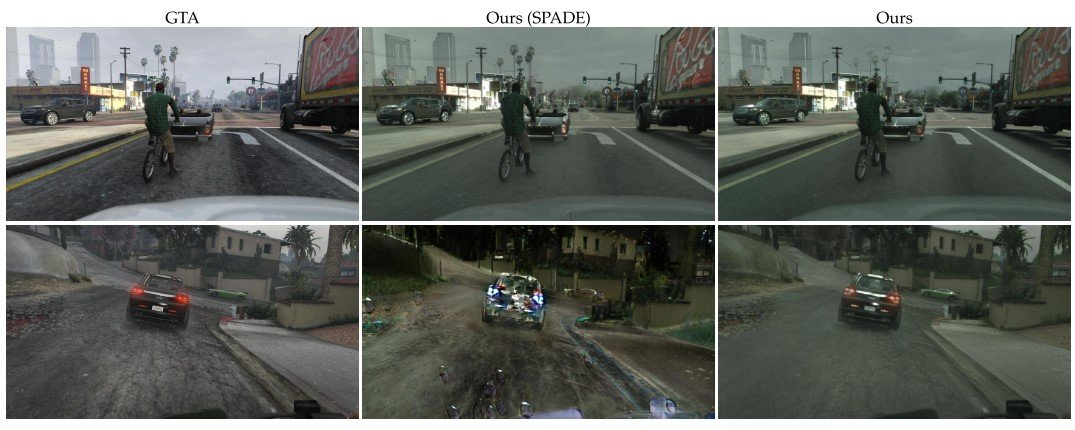Figure 4: Pre-training of encoder and decoder

The encoder $\xi$ consists of a feature extractor $\xi^{feat}$, two encoding heads $\xi^{content}$, and $\xi^{theme}$. The $\xi^{feat}$ consists of multiple convolutional layers such that the image $x$ is input and the output is passed to the two heads. The $\xi^{content}$ produces a $N x N$-dimensional $z^{content} \in \mathbb{R}^{N x N x D_1}$. On the other hand, $\xi^{theme}$ generates a single vector, $z^{theme} \in \mathbb{R}^{D_2}$, such that the theme of the output image is controllable. Collectively, these latent variables are denoted by $z = \{ z^{content}, z^{theme} \}$.

Next, the latent variable $z$ generated here is input to the decoder of StyleGAN, which can control the appearance of the generated image by using adaptive instance normalization (AdaIN) after each convolutional layer of the generator. AdaIN adapts the scaling and bias as well as the spatial location of the normalized feature map.

\begin{equation}AdaIN (m, \alpha, \gamma) = \mathcal{A}(m, \alpha, \gamma) = \alpha \frac{m - \mu(m)}{\sigma(m)} + \gamma \end{equation}

$m \in \mathbb{R}^{NxMx1}$ is the feature map and $\alpha, \gamma$ are the constants corresponding to the scaling and bias. By inputting this $z^{theme}$ into the MLP, we obtain the values of scaling and bias used in the AdaIN layer.

Then, from the shape of $z^{content}$, we can encode the content information corresponding to the grid position of $N x N$. Instead of the usual block input in StyleGAN, here we use $z^{content}$ as input.

Furthermore, we sample a new vector $v \in \mathbb{R}^{1x1xD}$ from the standard prior distribution to replace the grid position content. These innovations in encoding allow us to obtain detailed information in scenes with multiple objects since scaling and bias correspond to locations in space in the AdaIN layer. In addition, a multi-scale multi-patch discriminator is used as the discriminator, which can generate highly accurate images of more complex scenes. The loss function is the same as StyleGAN for adversarial loss, and the final one is $L_{pretrain} = L_{VAE} + L_{GAN}$.

As a simple experimental result, we found that the quality of the reconstruction is improved by reducing the value of $\beta$ used in the KL term, but we also found that the learned latent space is far from the prior distribution when the dynamics model has difficulty learning the dynamics. This means that $Z$ is overlearned to $X$, and learning transitions from an overlearned latent space is a future task.

### Dynamics Engine

After pre-training with the above encoder-decoder, the Dynamics Engine introduced here learns the transitions given the action $a_t$ at each step, based on the latent variables obtained with the encoder-decoder. In order to learn only the parameters of the Dynamics Engine, the parameters of the encoder and decoder are fixed. This makes it possible to extract the latent variables of the dataset in advance before training. Since the input is a latent variable, it is much faster than learning with images. In addition, we disentangle the content information obtained from the latent variable $Z^{content}$ into action-dependent and action-independent features.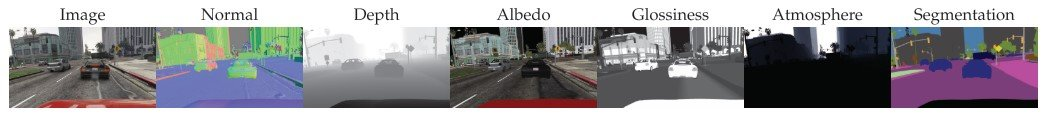Figure 5: Structure of the Dynamics Engine

In a 3D environment, the viewpoint changes according to the motion of the self-agent. Since this change occurs spatially naturally, we exercise a convolutional LSTM module to learn the spatial transitions at each time step.

We also add an LSTM module that takes only $z_t$ as input. This allows us to handle information that is independent of the action $a_t$.

Intuitively, $Z^{a_{indep}}$ is used to determine the style of the spatial tensor $Z^{a_{dep}}$ through the AdaIN layer. Since $Z^{A_{indep}}$ itself cannot provide actionable information, it cannot learn the next frame to seek. This structure allows us to disentangle action-dependent features such as the layout of the scene from action-independent features such as the type of object. Therefore, the engine only needs to consider the action-dependent features to learn dynamics. This is a better way to learn with smaller models.

Next is learning. In the training, we extend the training procedure of GameGAN in the latent space to train with adversarial loss and VAE loss. adversarial loss $L_{adv}$ consists of two networks ①latent discriminator ②temporal action-conditioned discriminator.

\begin{equation} L_{DE} = L_{adv} + L_{latent} + L_{action} + L_{KL} \end{equation}

We use $L_{adv}$ as hinge loss with adversarial loss. We also perform gradient regularization of $R_1$ to penalize the gradient of the discriminator for the true data. $L_{action}$ reconstructs the input action $a_{t-1}$ bypassing the temporal discriminator feature $z_{t, t-1}$ through a linear layer with action reconstruction loss, and calculates their mean squared error. In $L_{latent}$, the loss function measures whether the generated $z_t$ matches the input latent variable and is trained to reduce the KL penalty.

### Differentiable Simulation

Since DriveGAN is differentiable, it is possible to reproduce scenes and scenarios, even the actions of agents, by finding some of the factors that make up the video. We call this kind of simulation a differentiable simulation. Once the configurable parameters are found, the agent can use DriveGAN to re-stimulate different actions in the same scene, and since DriveGAN can also sample and change various components in the scene, it is possible to re-simulate the agent under different weather conditions and objects using the same scenario. The same scenario can be used to experiment with agents in different weather conditions and under different objects.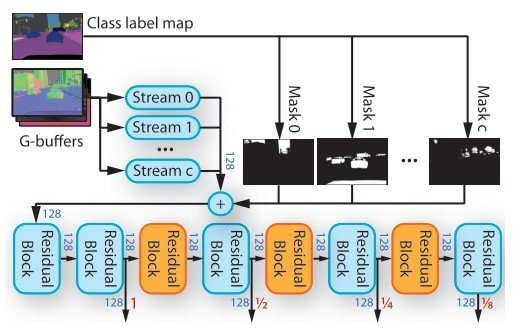Figure 6: Example of Differentiable simulation

The test of AGENT here is represented by a formula.

\begin{equation} \underset{a_{0 . . T-1}, \epsilon_{0 . . T-1}}{\operatorname{minimize}} \sum_{t=1}^{T}\left\|z_{t}-\hat{z}_{t}\right\|+\lambda_{1}\left\|a_{t}-a_{t-1}\right\|+\lambda_{2}\left\|\epsilon_{t}\right|| \end{equation}

The $\epsilon$ is a random variable that probabilistically generates future scenes within the simulation. The real-world video is $x_0, \ldots, x_T$, $z_t$ is the output of the model, $\hat z_t$ is a latent variable that encodes $x_t$ using an encoder, and $\lambda_1, lamda_2$ are hyperparameters to regularize. action. For consistency, we use the regularization terms of $a_t$ and $a_{t-1}$.

## Experiment and Evaluation

### Experimental setup

We use several datasets: the Carla simulator, which is a simulator for automated driving; the Gibson environment, which simulates a real-world indoor building environment with a physics engine and controllable virtual agents; and Real-World Driving (RWD), which records human driving in multiple cities and highways. The action is two-dimensional and consists of the agent's velocity and angular velocity.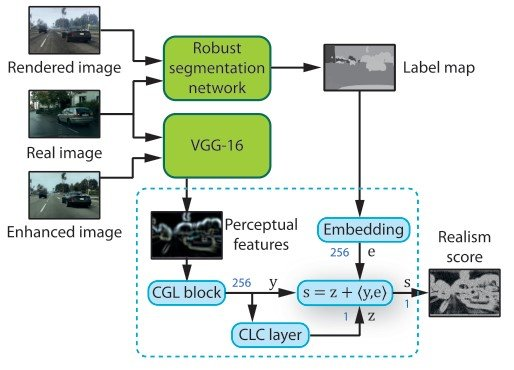Figure 7: Three sample data sets.

### qualitative evaluation

The qualitative aspects of the simulator are evaluated from two perspectives: one is whether the videos generated by the simulator are realistic and whether their distribution matches the distribution of real videos, and the other is whether the ACTION is realistically acceptable. To measure these, we have created two evaluation methods to evaluate them.

The baseline models are Action-RNN, a simple action-following RNN model, Stochastic Adversarial Video Prediction (SAVP ), GameGAN, and a mixture distribution network trained with the base RNN World Model. Here are the results.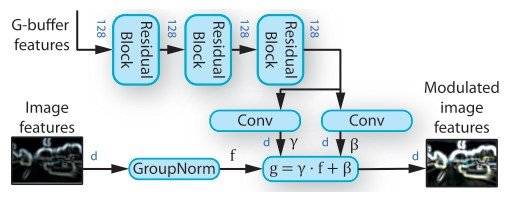Figure 8: Comparison of baseline modelsFigure 9: Example of a generated image

The first evaluation method is to measure the distance between ground truth and the distribution of generated videos using Fr ́echet VideoDistance (FVD ).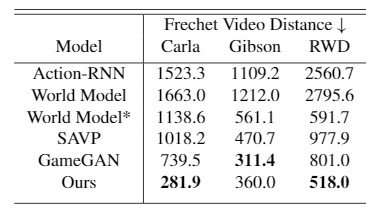Table 1: Results of FVD

The results are also of high quality in human evaluations.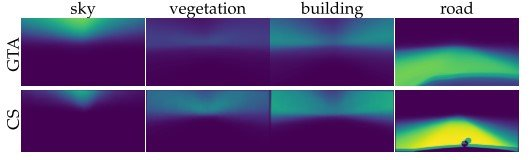Figure 10: Evaluation by humans

The second is a measure of action consistency, where two images extracted from real-world videos are trained with a CNN to predict an action that represents a transition in the images. The model is then trained to reduce the mean squared error between the input action and the predicted one.Table 2: Results of Action Prediction

### Differentiable Simulation

DriveGAN allows you to control the simulation using latent variables $z^{a_{dep}}, z^{a_{indep}}, z^{content}, z^{theme}$ sampled from the prior distribution.

In the following figure, the background color and weather can be changed by sampling $Z^{theme}$ and changing it.Figure 11: Changes in weather and background by theme.

By sampling different $Z^{A_{DEP}}$, we can change the interior as follows.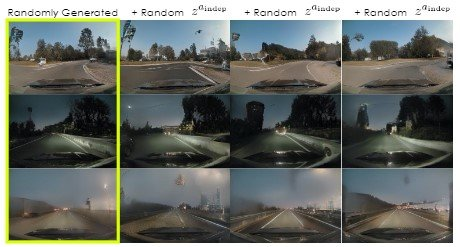Fig. 12: Change of objects in the scene by updating $Z^A_{INDEP}$.

Also, $Z^{content}$ can change the components of the corresponding cell by varying each grid cell in the space tensor.Figure 13: Random sampling of grid cells to change cell components.

## at the end

DriveGAN proposed a controllable, high-quality simulation that uses encoder and image GAN to generate a latent space in which the dynamics engine can learn transitions between frames. We have succeeded in unsupervised disentangling and sampling different components of the scene. This allows the user to interactively edit the scene during the simulation and create unique scenarios. In the future, this research is expected to create an environment in which differentiable simulations can be used for costly experiments such as robotics and automated driving.

Also, once the code is released now (at the time of writing: June 7, 2021), I think we'll be able to use the simulator with the following UI, which should be fun!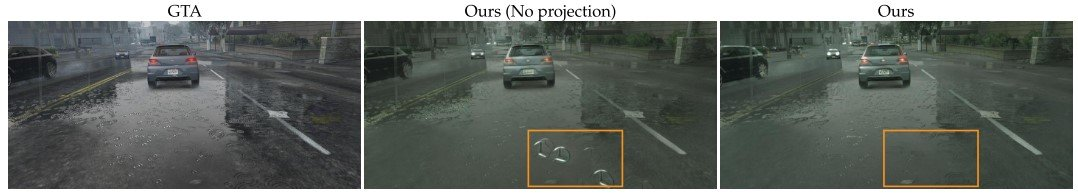Figure 14: UI of the Drive GAN simulator

If you have any suggestions for improvement of the content of the article,
please contact the AI-SCHOLAR editorial team through the contact form.